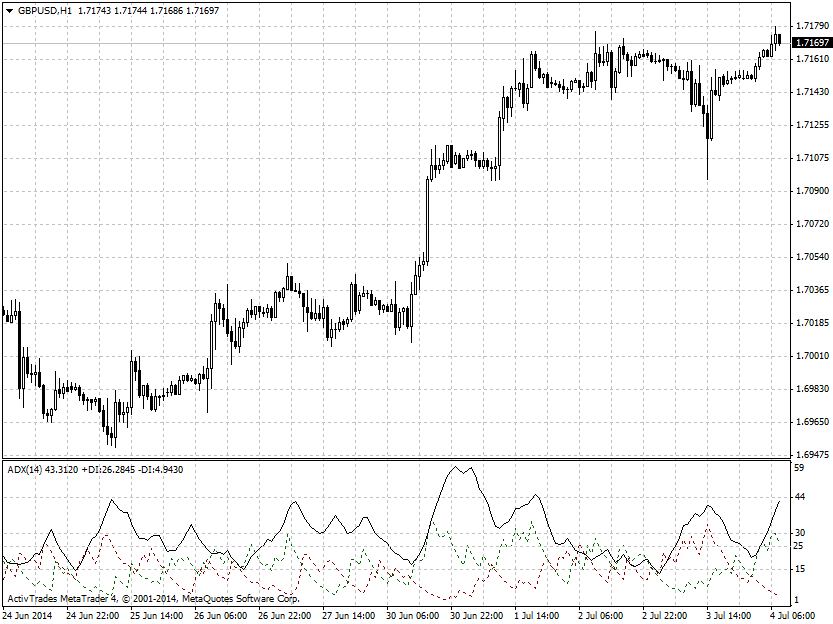# forex software

Create and Test Forex Strategies

forex software

trading:adx

# Average Directional Index (ADX)

ADX was developed by J. Welles Wilder as an indicator of the strength of the trend. It is plotted along with two other indicators Plus Directional Indicator (+DI) and Minus Directional Indicator (-DI) which define the trend direction. The calculation of the components of the ADX is as follows:

1st step

• Up Move = last high − previous high
• Down Move = last low − previous low
• if Up Move > Down Move and Up Move > 0, then +DM = Up Move, else +DM = 0
• if Down Move > Up Move and Down Move > 0, then −DM = Down Move, else −DM = 0

2nd step

• +DI = 100 times EMA of +DM divided by ATR
• −DI = 100 times EMA of −DM divided by ATR

3rd step

• ADX = 100 times EMA of the absolute value of (+DI − −DI) divided by (+DI + −DI)

ADX is used to measure the strength or weakness of a trend, but not the actual direction. According to Wilder, a strong trend is present when ADX is above 25 and no trend is present when below 20. The direction of the trend is defined by +DI and -DI. The bulls control the market when +DI is greater than - DI, while the bears have the edge when - DI is greater. Crosses of these directional indicators can be combined with ADX for generating of buy and sell signals.